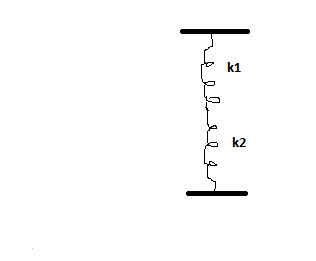# Two springs have spring constants k_1 \space \textbf{and} \space k_2, k_2 is greater than k_1....

## Question:

Two springs have spring constants {eq}k_1 \space \textbf{and} \space k_2, k_2 > k_1 {/eq}. Connected as shown in figure, they act like one spring. Compute the spring {eq}k {/eq} constant of the combination. Which is correct statement for {eq}k {/eq}? a) {eq}k < k_1 {/eq} b) {eq}k = k_1 {/eq} c) {eq}k_1 < k < k_2 {/eq} d) {eq}k = k_2 {/eq} e) {eq}k > k_2. {/eq}## Springs in Series

If springs are connected in series then the reciprocal of the effective spring constant is equal to the reciprocals of the spring constants of the individual springs

{eq}\dfrac{1}{k} = \dfrac{1}{k_{1}} + \dfrac{1}{k_{2}} {/eq}

Where k is the effective spring constant and {eq}k_{1} , k_{2} {/eq} are spring constants of individual springs.

## Answer and Explanation:

Become a Study.com member to unlock this answer! Create your account

The two springs shown in the figure are in series

Hence their effective spring constant is

{eq}\dfrac{1}{k} = \dfrac{1}{k_{1}} +...

See full answer below.

#### Learn more about this topic:Practice Applying Spring Constant Formulas

from

Chapter 17 / Lesson 11
3.4K

In this lesson, you'll have the chance to practice using the spring constant formula. The lesson includes four problems of medium difficulty involving a variety of real-life applications.